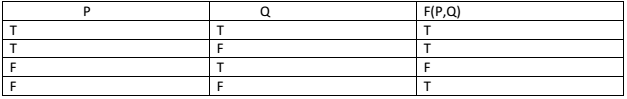# GATE | GATE 2017 MOCK II | Question 37

Given F is an expression of P,Q. Derive the expression F(P,Q) from the truth table given below.(A) (PQ)’
(B) P + Q’
(C) P + Q
(D) (P + Q)’

Explanation: From the truth table, the value of F can be written as:
=> F = PQ + PQ’ + P’Q’
=> P(Q+Q’)+P’Q’
=> P+P’Q’
=> (P+Q’)(P+P’)
=> (P+Q’)T
=> (P+Q’)